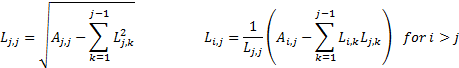/

### Enterprise Risk Management Formula Book 6. Monte Carlo methods

6.1          Creation of normal random variables

Box-Muller: if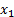and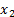are independent standard uniform random variables, i.e. come from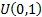and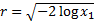then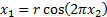and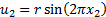are independent standard normal random variables.

Polar method: if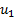and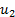are independent random variables from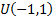,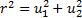and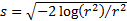thenand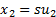are independent standard normal random variables.

If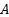has real entries, is symmetric and is positive definite then it can be decomposed as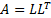where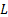is a lower triangular matrix with strictly positive diagonal entries and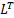is its transpose. The entries ofare: